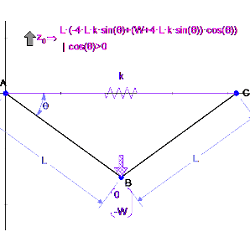# Explore Statics Examples

Add force elements to your model, and you can measure resultant forces and torques.#### Force and Spring Equilibrium

An expression is derived which, when solved will yield the equilibrium position of a spring loaded mechanism under an applied force.#### Crank Slider Torque

An app which derives the torque in a crank slider mechanism due to a given applied force.#### Forces in a Simple Structure

We show how body forces are computed as reactions in constraints. We also show how to compute reactions at pins.